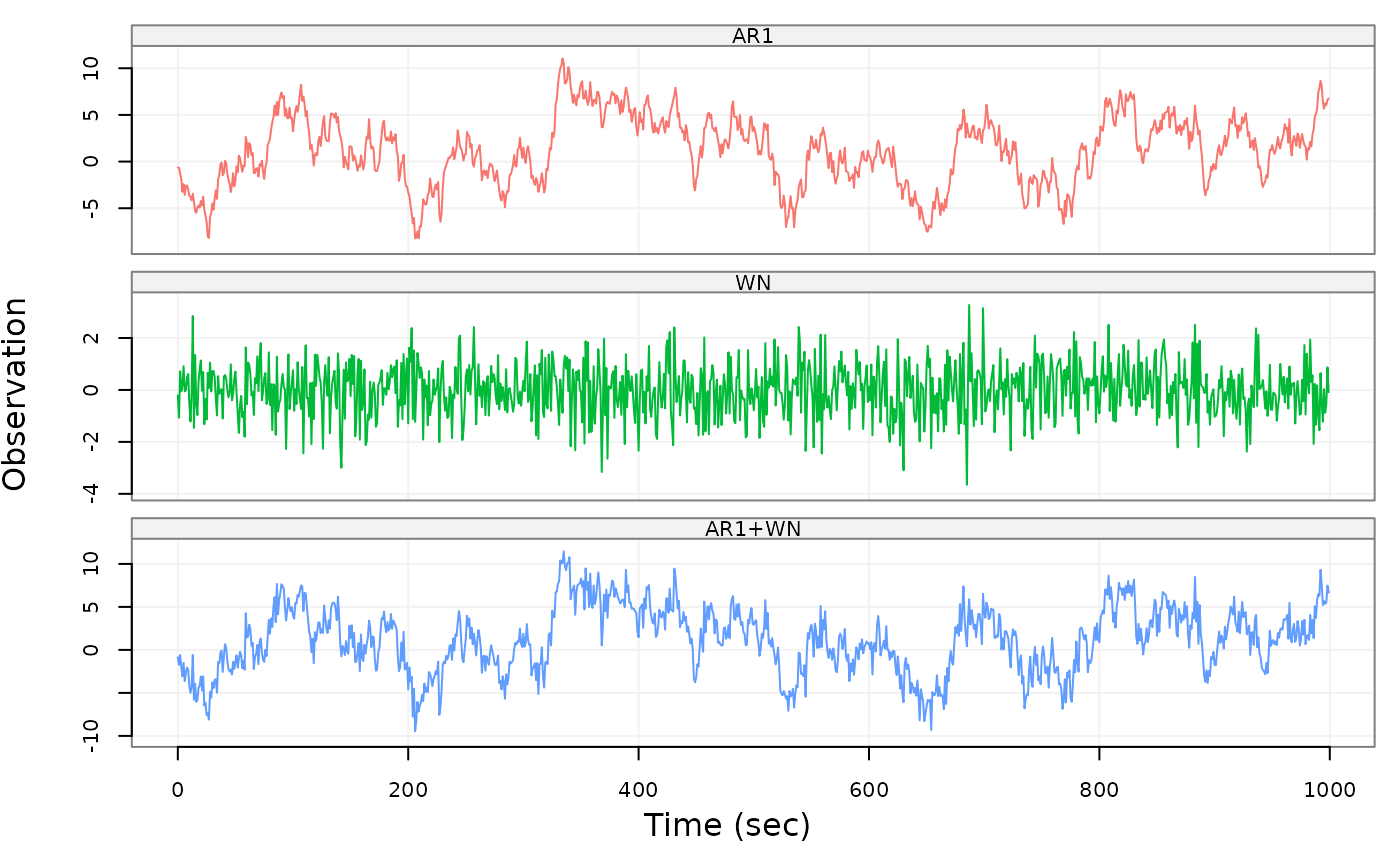Create a lts object based on a supplied matrix or data frame. The latent time series is obtained by the sum of underlying time series.

lts(
data,
start = 0,
end = NULL,
freq = 1,
unit_ts = NULL,
unit_time = NULL,
name_ts = NULL,
name_time = NULL,
process = NULL
)

## Arguments

data

A multiple-column matrix or data.frame. It must contain at least 3 columns of which the last represents the latent time series obtained through the sum of the previous columns.

start

A numeric that provides the time of the first observation.

end

A numeric that provides the time of the last observation.

freq

A numeric that provides the rate/frequency at which the time series is sampled. The default value is 1.

unit_ts

A string that contains the unit of measure of the time series. The default value is NULL.

unit_time

A string that contains the unit of measure of the time. The default value is NULL.

name_ts

A string that provides an identifier for the time series data. Default value is NULL.

name_time

A string that provides an identifier for the time. Default value is NULL.

process

A vector that contains model names of each column in the data object where the last name is the sum of the previous names.

## Value

A lts object

## Author

Wenchao Yang and Justin Lee

## Examples

model1 = AR1(phi = .99, sigma2 = 1)
model2 = WN(sigma2 = 1)
col1 = gen_gts(1000, model1)
col2 = gen_gts(1000, model2)
testMat = cbind(col1, col2, col1+col2)
testLts = lts(testMat, unit_time = 'sec', process = c('AR1', 'WN', 'AR1+WN'))
plot(testLts)